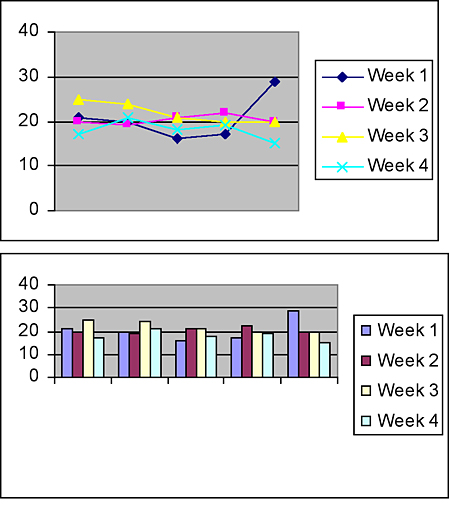# Stage 4 data – data analysis and evaluation

## Strategy

Students can:

• find measures of location (mean, mode and median) for small sets of data
• use measures of location and range to analyse data that is displayed in a frequency table, stem and leaf plot or dot plot
• use spreadsheets to tabulate and graph data.

## Activities to support the strategy

### Activity 1 – measures of location and range

1. Provide your students with an example data set showing the results of a survey and revise the terms range, mode, median and mean. Use this link to find information, activities and worksheets for revising how to calculate the mean, median, mode and range Mathdrills.
2. Students analyse the data set and explore the effect of an outlier (a statistical observation that is markedly different in value from the others of the sample) on the measures of location.
3. Students discuss the appropriateness of using the mean and the median when analysing data.

A small group of students in a Year 8 class was surveyed on how many text messages they sent during a particular week. The survey results are displayed in a stem and leaf plot shown.Calculate the following:

(a) Range

(b) Mode

(c) Median

(d) Mean

Which measure would be the best indicator of typical number of text messages that a student from this group sent during the week - the mean or the median? Is the mode suitable as a measure of the typical number of text messages sent?

The score of 75 in the data set is a good example of an outlier – an extreme value far beyond or below the other numbers in a data set. Suppose the outlier is removed from the set of scores. Explain how this would affect the mean, the median, the mode and the range.

The group surveyed was chosen from 120 students in Year 8. How many students from this Year 8 group would you expect to send less than the modal number of text messages for this group?

Perform a class survey to explore the number of text messages sent in a week and to see if there are differences between the number of text messages sent by boys and girls. Display this information in a back-to-back stem and leaf plot.

Extend the survey to other students in your year and compare your findings. For example, is the mean number of text messages sent by boys in your class the same as that for boys in your year group?

Would you expect the results to be the same for older year groups? How would you gather and organise your data?

### Activity 2 – daily temperature

1. Provide students with a range of small sets of data, e.g. number of students in each cohort or in each class in the school. Introduce the terms mean. Ask students to define each term and how it is identified or calculated.
2. Students compare two different sets of data by finding the mean, mode, median and range of both sets. Data could be obtained from conducting surveys, e.g. canteen foods, preferred music.
3. Students analyse the data and draw conclusions using the mean, mode, median and range.
4. Students record the daily outdoor temperature over a month. Students then calculate the average temperature and submit their data in a two-way table using Excel. Provide students with this task sheet as a guide.

To record and analyse the local temperature for one month and compare to the official temperature recordings.

#### Equipment required:

• computers with internet access
• thermometer
• notebook and pencils

#### Procedure:

• Record daily temperatures for a month using a thermometer
• Calculate the average weekly temperature
• Calculate the average monthly temperature
• Summarise and record raw data in a two-way table in an Excel document
• Record the official temperature from the website bom.gov.au
• Record and save daily data to your class intranet file.

Each day, your recorded information needs to include:

• time
• date
• temperature in degrees Celsius
• View/print (PDF 240.79KB)

### Recording the daily temperature

Use the table below to record the daily temperatures. When measuring the temperature, don't forget the following:

• Measure the temperature between 11 a.m. and 1 p.m.
• Measure the temperature in the shade.
• Hold the thermometer close to eye level; it should never be on the ground.
• Do not allow rain or snow to fall on the thermometer.
• Record the temperature each day in degrees Celsius.Copy the information into Microsoft Excel

5. Show students how to use Excel to calculate averages and represent the temperature data in different graphical forms.

Students open an Excel spreadsheet and type:

• the data in the correct cell

Find the average temperature for a week

Students use Excel to answer these questions:

• What was the average temperature for the week?
• What was the average temperature for the month?

By going to 'Insert' on the Main menu and clicking 'Chart', the students can follow the Chart Wizard and represent their temperature data in different graphical forms, e.g.Students write a summary of their findings which compares their recordings to the official weather recordings from the Bureau of Meteorology.

6. Students investigate a real life situation of their choice, which involves a range of scores and averages. Suggested ideas:

• the average price of a second-hand car
• the average house price for your local area
• the average shoe size of a group of people.

Students present the results of their investigation, which must include:

• tables and/or graphs
• a scale or key if presenting a graph
• a summary of the findings and what they have learnt from the investigation.

## References

### NSW syllabus

MA4-20SP: Analyses single sets of data using measures of location, and range.

• Science